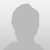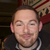+1``` <wire> <main> <panel name="list-container" alias="HIGH" width="100%" height="100%"> <panel name="yes" alias="YES" width="100" height="100" align="center" valign="center" onclickup="grow" background="ff0000"> <web name="hi" alias="HI" width="100%" height="100%" align="center" url="about://blank" pagetofit="yes" alpha="0"></web> </panel> </panel> </main> <actions> <action name="grow"> <sync loop="no" startdelay="0"> <scale time="1" width="100%" height="100%" align="center" valign="center" target="YES" /> <load url="http://espn.com" target="HI" /> <alpha value="1" time="0" target="HI" /> </sync> </action> </actions> </wire> ```